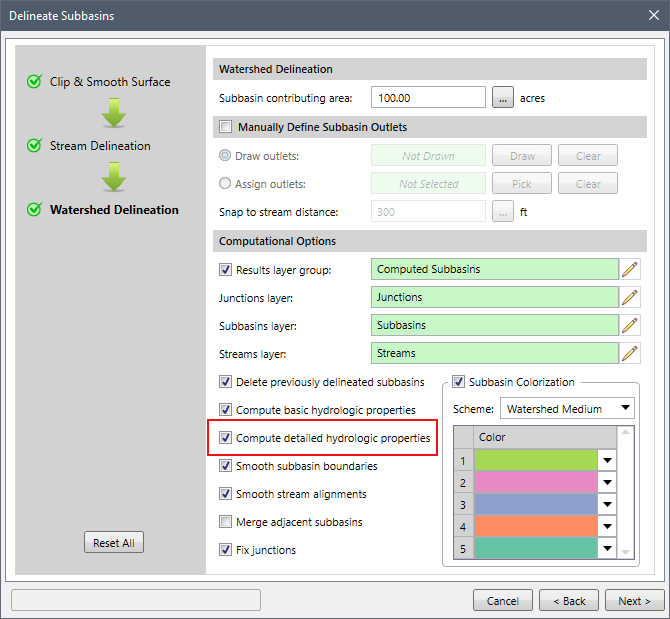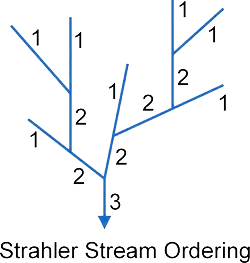1. Home
2. Knowledge Base
3. GeoHECHMS
4. Hydrologic Properties

# Hydrologic Properties

## Basin Hydrologic Properties Computation

After the subbasins have been delineated, the software computes the hydrologic properties (attributes) for each subbasin. The GIS hydrologic attribute variables are represented by square brackets (shown below), allowing the user to run queries on this data.

### Basic Properties

The following hydrologic properties are always computed for subbasins, regardless of the detailed hydrologic properties checkbox option.

• Basin area [BASINAREA]
Area of the drainage basin.
• Basin perimeter [BASINPERIM]
Perimeter length the drainage basin boundary (divide).
• Overland flow slope (mean) [OVRFLSLAVG]
Computed by summing the slope of each cell within the subbasin, and then dividing by the number of cells within the subbasin. Reported as a decimal percent slope.
• Overland flow slope (maximum) [OVRFLSLMAX]
Computed by reviewing the slope of each cell within the drainage subbasin and returning the maximum value. Reported as a decimal percent slope.
• Stream slope (average) [STRMSPLAVG]
Average slope of all streams within the subbasin, from the top of each stream to where the stream exits the subbasin at the outlet. If more than one stream exists within a subbasin, then this value represents the average slope of the stream with the maximum flow length (i.e., STREAMFLOWLEN). Reported as a decimal percent slope.
• Stream slope (maximum) [STRMSPLMAX]
Maximum slope of all streams within the subbasin, from the top of each stream to where the stream exits the subbasin at the outlet. If more than one stream exists within a subbasin, then this value represents the maximum slope of the stream with the maximum flow length (i.e., STREAMFLOWLEN). Reported as a decimal percent slope.
• Stream length (maximum) [STRMLENMAX]
Computed by determining the flow distance traveled from the top of each stream within the subbasin and to where the stream exits the basin at the outlet. If more than one stream exists within a subbasin, then this value represents the maximum flow distance of the evaluated streams.
• Stream length (total) [STREAMLEN]
Computed by determining the flow distance traveled from the top of each stream within the subbasin and to where the stream exits the subbasin at the outlet. If more than one stream exists within a subbasin, then this value represents the summation of all flow distances of the evaluated streams.

The following hydrologic properties are only computed for the subbasins when you select the Compute detailed hydrologic properties checkbox as shown below.• Basin azimuth [BSNAZIMUTH]
The compass direction of a straight line from the drainage subbasin outlet to the farthest point on the subbasin parameter. Reported as decimal degrees, measured clockwise from North at 0°.
• Basin compactness ratio [BSNCOMPRAT]
Computed as the ratio of the subbasin perimeter to the circumference of a circle of equal area (i.e., BASINPERIM / CIRCLEPERIM).
• Basin elevation (mean) [BSNELVMEAN]
Mean elevation within the subbasin, computed by adding up the elevations of each of the cells contained within the subbasin and then dividing by the number of cells within the subbasin.
• Basin elevation (maximum) [BSNELEVMAX]
Computed by reviewing the elevation of each cell within the subbasin and returning the maximum value.
• Basin elevation (minimum) [BSNELEVMIN]
Elevation of the cell at the subbasin cell.
• Basin elevation (relief) [BSNELEVRLF]
Elevation change contained within the subbasin, computed by BSNELEVMAX – BSNELEVMIN.
• Basin elevation (relative relief) [BSNELEVREL]
Normalized subbasin relief, computed by BSNELEVRLF / BASINPERIM.
• Basin length [BSNLENGTH]
Computed by measuring the distance in a straight line from the subbasin outlet to the farthest point on the subbasin parameter. Sometimes referred to as valley length.
• Basin shape factor [BSNSHPFCTR]
Computed by dividing basin length by basin width. Sometimes referred to as basin slenderness ratio.
• Basin width [BASINWIDTH]
Computed by dividing basin area by subbasin length. Sometimes referred to as valley width.
• Overland flow distance [OVRFLWDIST]
Computed by averaging the overland distance traveled from the centroid of each cell within the subbasin to the nearest stream within the subbasin.
• Percent North facing [PRCNTNORTH]
The percentage of the subbasin whose aspect is directed North, where North is defined as the positive Y direction. Reported as a decimal percent.
• Percent South facing [PRCNTSOUTH]
The percentage of the subbasin whose aspect is directed South, where South is defined as the negative Y direction. Reported as a decimal percent.
• Stream density [STRMDENSTY]
Computed as the total stream length divided by the subbasin area (i.e., STREAMLEN / BASINAREA). Reported as miles per mile² or km per km².
• Stream order (Shreve method) [STRMORSHRV]
Stream order computed by Shreve method of the stream at the subbasin outlet. This method of stream ordering by magnitude, proposed by Shreve in 1967. All links with no tributaries are assigned a magnitude (order) of one. Magnitudes are additive downslope. When two links intersect, their magnitudes are added and assigned to the downslope link.• Stream order (Strahler method) [STRMORSTHR]
Stream order computed by Strahler method of the stream at the subbasin outlet. This method of stream ordering was proposed by Robert E. Horton (1945) and Arthur Newell Strahler (1952, 1957). All streams with no tributaries are assigned a base order of 1 and the stream order only increases when streams of the same order intersect. Therefore, the intersection of a first-order and second-order link will remain a second-order stream, rather than create a third-order stream.• Stream first order [STRMFRORDR]
Number of first order stream segments contained within the subbasin. This value is the same for either the Strahler or Shreve stream ordering method.
• Stream sinuosity [STRMSNOSTY]
Computed by maximum stream length within the subbasin divided by the subbasin length (STREAMLEN / BASINLEN).
• Total flow length [TLFLOWLEN]
Total flow distance within the subbasin, including both overland and stream flow.
• Total flow slope (average) [TLFLSLPAVG]
Average flow slope within the subbasin, including both overland and stream flow. Reported as a decimal percent slope.
• Total flow slope (maximum) [TLFLSLPMAX]
Maximum flow slope within the subbasin, including both overland and stream flow. Reported as a decimal percent slope.
• Total flow slope ratio [TLFLSLPRAT]
Computed as the average stream slope divided by the average overland flow slope.
• Total stream length [TLSTRMLEN]
Total length of all streams within the subbasin.

## Stream Hydrologic Properties Computation

After the subbasins have been delineated, the software computes the hydrologic properties (attributes) for each stream. The hydrologic attribute variables are represented by square brackets (shown below), allowing the user to run queries on this data.

### Basic Properties

The following hydrologic properties are always computed for the streams, regardless of the detailed hydrologic properties checkbox option.

• Stream segment length [SSTRMLEN]
Length of the stream segment.
• Stream segment slope (average) [SSTRMSLAVG]
Average slope of the stream segment. Reported as a decimal percent slope.
• Stream segment slope (maximum) [SSTRMSLMAX]
Maximum slope of the stream segment. Reported as a decimal percent slope.

The following hydrologic properties are only computed for the streams when you select the Compute detailed hydrologic properties checkbox as shown below.• Stream segment elevation (average) [SSTRMELAVG]
Average elevation of the stream segment, computed by averaging the maximum and minimum elevation of the stream segment.
• Stream segment elevation (maximum) [SSTRMELMAX]
Maximum elevation of the stream segment.
• Stream segment elevation (minimum) [SSTRMELMIN]
Minimum elevation of the stream segment.
• Stream segment elevation (relief) [SSTRMELREL]
Elevation change along the stream segment, computed by SSTRMELMAX – SSTRMELMIN.
• Stream segment order (Shreve method) [SSTRMORSHR]
Stream order computed by Shreve method of the stream segment. This method of stream ordering by magnitude, proposed by Shreve in 1967. All links with no tributaries are assigned a magnitude (order) of one. Magnitudes are additive downslope. When two links intersect, their magnitudes are added and assigned to the downslope link.• Stream segment order (Strahler method) [SSTRMORSTR]
Stream order computed by Strahler method of the stream segment. This method of stream ordering was proposed by Robert E. Horton (1945) and Arthur Newell Strahler (1952, 1957). All streams with no tributaries are assigned a base order of 1 and the stream order only increases when streams of the same order intersect. Therefore, the intersection of a first-order and second-order link will remain a second-order stream, rather than create a third-order stream.